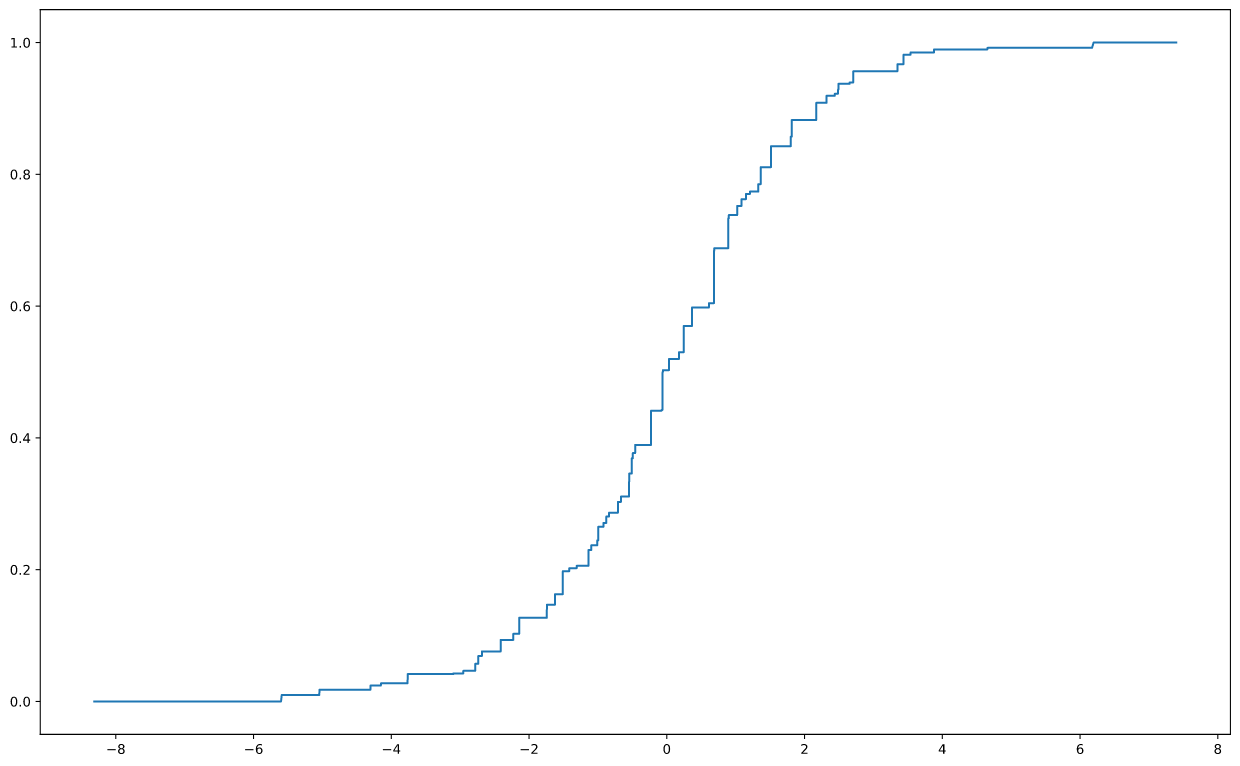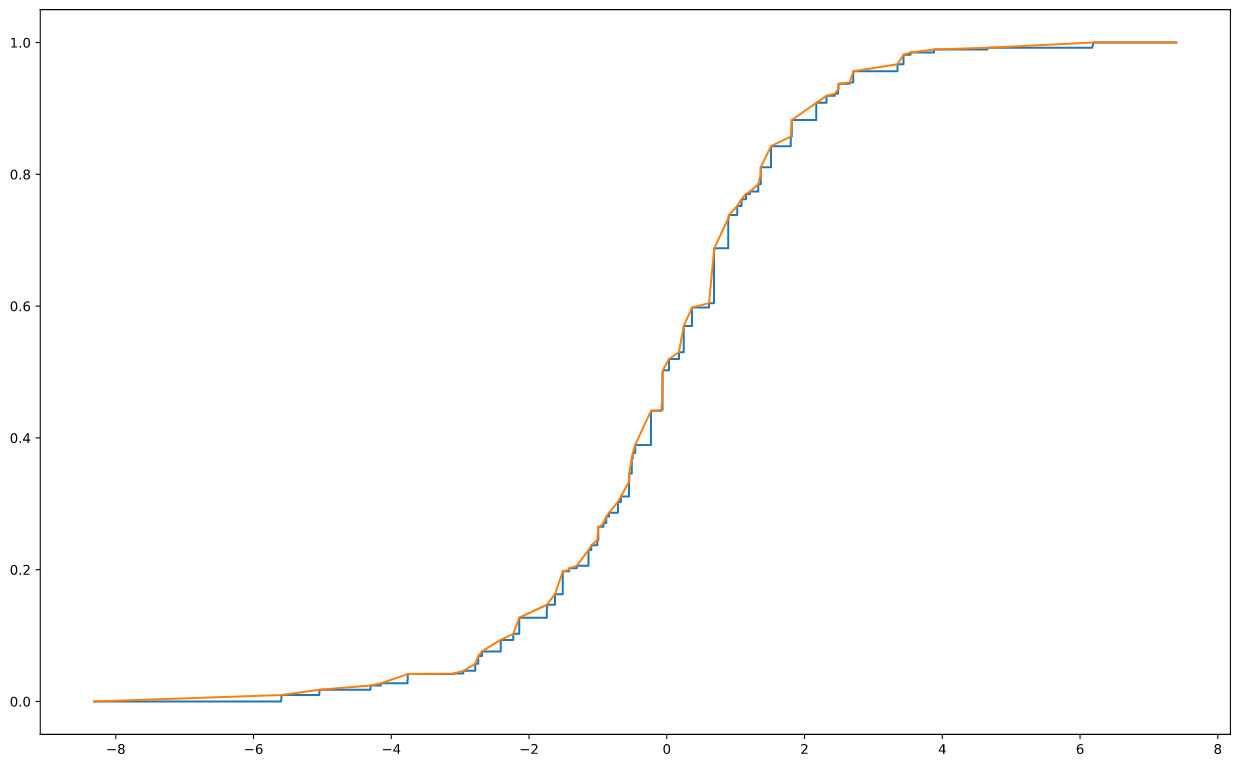{{ message }}
/ scikit-learn Public

# Isotonic calibration changes rank-based test metrics values#16321

Open
opened this issue Jan 30, 2020 · 13 comments
Open

# Isotonic calibration changes rank-based test metrics values#16321

opened this issue Jan 30, 2020 · 13 comments
Labels

###dsleo commented Jan 30, 2020

[As discussed with @ogrisel]

#### Describe the bug

Using isotonic calibration changes metrics values. This is because it is a non-strictly monotonic calibration. Sigmoid calibration being strictly monotonic doesn't suffer from this.

#### Steps/Code to Reproduce

Here is quick example where we split in three train/calibration/test sets and compare ROC AUC on the test set before and after calibrating for both isotonic and sigmoid..

```import numpy as np
from sklearn import datasets
from sklearn.model_selection import train_test_split
from sklearn.linear_model import LogisticRegression
from sklearn.calibration import CalibratedClassifierCV
from sklearn.metrics import roc_auc_score

X, y = datasets.make_classification(n_samples=100000, n_features=20,
n_informative=18, n_redundant=0,
random_state=42)

X_train, X_, y_train, y_ = train_test_split(X, y, test_size=0.5,
random_state=42)
X_test, X_calib, y_test, y_calib = train_test_split(X_, y_, test_size = 0.5,
random_state = 42)

clf = LogisticRegression(C=1.)
clf.fit(X_train, y_train)

y_pred = clf.predict_proba(X_test)
print(roc_auc_score(y_test, y_pred[:,1]))```

The ROC AUC is then: 0.88368

```isotonic = CalibratedClassifierCV(clf, method='isotonic', cv='prefit')
isotonic.fit(X_calib, y_calib)

y_pred_calib = isotonic.predict_proba(X_test)
print(roc_auc_score(y_test, y_pred_calib[:,1]))```

After isotonic calibration, the ROC AUC becomes: 0.88338

```isotonic = CalibratedClassifierCV(clf, method='sigmoid', cv='prefit')
isotonic.fit(X_calib, y_calib)

y_pred_calib = isotonic.predict_proba(X_test)
print(roc_auc_score(y_test, y_pred_calib[:,1]))```

As expected for sigmoid calibration, the ROC AUC is constant. 0.88368

#### Versions

System:
python: 3.8.1 | packaged by conda-forge | (default, Jan 29 2020, 15:06:10) [Clang 9.0.1 ]
executable: /Users/leodreyfusschmidt/opt/miniconda2/envs/isotonic/bin/python
machine: macOS-10.13.4-x86_64-i386-64bit

Python dependencies:
pip: 20.0.2
setuptools: 45.1.0.post20200119
sklearn: 0.22.1
numpy: 1.16.5
scipy: 1.4.1
Cython: None
pandas: None
matplotlib: 3.1.2
joblib: 0.14.1

Built with OpenMP: True

###ogrisel commented Jan 31, 2020 • edited

 Thank you very much @dsleo for the detailed report. I started from your code and pushed the analysis further. If one introspect the calibrator, on can observe the following: ```y_pred_calib = isotonic.predict_proba(X_test) print(roc_auc_score(y_test, y_pred_calib[:, 1])) calibrator = isotonic.calibrated_classifiers_.calibrators_ import matplotlib.pyplot as plt plt.figure(figsize=(16, 10)) plt.plot(calibrator._necessary_X_, calibrator._necessary_y_)```Note that this kind of plot is very interesting and there is another related PR (#16289) to make it possible to expose those thresholds for inspection reasons here. This is a bit weird to have a constant step wise function to map `y_raw_pred` to `y_cal_pred`. Furthermore a close look at the (x, y) pairs of thresholds look as follows (for the first 10 pairs): ```for x, y in zip(calibrator._necessary_X_[:10], calibrator._necessary_y_[:10]): print(x, y)``` ``````-8.31123478939124 0.0 -5.600958281618134 0.0 -5.590794325886634 0.00980392156862745 -5.044899575456449 0.00980392156862745 -5.042974497498811 0.017804154302670624 -4.303066186168206 0.017804154302670624 -4.301859876807849 0.024193548387096774 -4.151532225028019 0.024193548387096774 -4.149327735774874 0.027548209366391185 -3.763637782629291 0.027548209366391185 `````` The y values are monotonic (but not strictly) but what is weird is that the x values are not even monotonic and go a bit back in time at each step. This is a bit fishy but fair enough, once we build the `scipy.interpolation.interp1d` function on the threshold in `IsotonicRegression` these artifacts can be ignored. I don't know why we want to use such a piece-wise constant mapping. One could instead use a piece-wise linear mapping that would be trivially strictly monotonic. Here is a quick hack to show that this would trivially fix the issue when using isotonic calibration for classifier calibration: ```X_thresholds_fixed = np.concatenate((calibrator._necessary_X_[::2], calibrator._necessary_X_[-1:])) y_thresholds_fixed = np.concatenate((calibrator._necessary_y_[::2], calibrator._necessary_y_[-1:])) plt.figure(figsize=(16, 10)) plt.plot(calibrator._necessary_X_, calibrator._necessary_y_) plt.plot(X_thresholds_fixed, y_thresholds_fixed)```Linearly interpolating using those thresholds makes the mapping strictly monotonic and the ROC-AUC of the original model is recovered: ```calibrator._build_f(X_thresholds_fixed, y_thresholds_fixed) y_pred_calib = isotonic.predict_proba(X_test) print(roc_auc_score(y_test, y_pred[:, 1])) print(roc_auc_score(y_test, y_pred_calib[:, 1]))``` ``````0.8836876399954915 0.8836876399954915 `````` Instead of the max values of each steps, one could have used mid-points. This could be explored in a fix in `IsotonicRegression` itself (maybe as a new option to keep the piece-wise constant mapping as the default behavior for backward compat reasons).

###ogrisel commented Feb 2, 2020 • edited

 The above analysis is wrong: I made wrong expectations on the structure of the steps. They are not always exact steps. There are already piece-wise linear components in the default prediction function. Still it would be nice to have an option to make it possible to enforce a strict monotonicity option, maybe by adding a small eps on one of the edges whenever y is constant on a segment.changed the title Isotonic calibration changes test metrics values Isotonic calibration changes rank-based test metrics values Feb 3, 2020

###ogrisel commented Feb 3, 2020

 Metrics that should not be impacted by Isotonic Calibration but are: ROC AUC Average Precision NDCG...

###lucyleeow commented Jun 23, 2020

 Linearly interpolating using those thresholds makes the mapping strictly monotonic and the ROC-AUC of the original model is recovered: This would solve the problem but we would have to make clear that we are not strictly doing isotonic regression. I had a look at what R does. While the core stats package and a number of external packages implement isotonic regression but none seem to deal with this issue. There is also few mentions of this in literature (that I could fine). 'Predicting accurate probabilities with a ranking loss' mentions: Observe that isotonic regression preserves the ordering of the input model’s scores, although potentially introducing ties i.e. f(ŝ(·)) is not injective. To break ties on training examples, we may simply refer to the corresponding original model’s scores. This would be a simple way to ensure rank stays consistent and we would still strictly be using isotonic regression but the API would be difficult to work out. The only other paper I found that touches on this 'Smooth Isotonic Regression: A New Method to Calibrate Predictive Models'. Their method would solve the rank problem but I think it is too complex for our purposes. Summary of their method: Obtain f* = argminf∑i (ci− f(pi))2, subject to f(pi) ≤ f(pi+1), ∀i (Isotonic Regression). Sample s points from f(γ), γ ∈ (0, 1), one point per flat region of f(·). Denote samples as P′, and their corresponding class labels as C′. Construct a Piecewise Cubic Hermite Interpolating Polynomial function t(f(P′), C′)) to obtain a monotone smoothing spline as the final transformation function for calibration.

###NicolasHug commented Jun 24, 2020

 Should we just add a note in the UG, something like It is generally expected that calibration does not affect ranking metrics such as ROC-AUC or Average Prevision. However, these metrics might differ after calibration when using method='isotonic' since isotonic regression introduces ties in the predicted probabilities. Using method='logistic' will not suffer from this because the sigmoid is strictly monotonic. Slightly related, it would be interesting to discuss in the UG when one might prefer sigmoid vs isotonic. I personally have no idea.

###lucyleeow commented Jun 24, 2020

 Slightly related, it would be interesting to discuss in the UG when one might prefer sigmoid vs isotonic. I personally have no idea. I am happy to add this to the doc. This was explained in some of the papers I looked at.

###NicolasHug commented Jun 24, 2020

 please ping me on the PR :)

###thomasjpfan commented Jun 24, 2020

 This would be a simple way to ensure rank stays consistent and we would still strictly be using isotonic regression but the API would be difficult to work out. I am curious if this can be worked into our `IsotonicRegression`. We can have it as an option if it adds too much computational cost.

###ogrisel commented Jun 25, 2020 • edited

 it would be interesting to discuss in the UG when one might prefer sigmoid vs isotonic. I personally have no idea. sigmoid is parametric and strongly biased (assume the calibration curve of the un-calibrated model has a sigmoid shape) while isotonic calibration is non-parametric and does not make this assumption. In general, the non-parametric calibration would tend to overfit on dataset with a small number of samples while the parametric sigmoid calibration can underfit if the assumption is wrong. TL;DR: sigmpoid on small datasets, isotonic on large datasets.

###ogrisel commented Jun 25, 2020

 I had a look at what R does. While the core stats package and a number of external packages implement isotonic regression but none seem to deal with this issue. Not all application of isotonic regression are for probabilistic calibration of classifiers. For the calibration use case it might make sense to further impose strict monotonicity. This could be a non-default option for the `IsotonicRegression` class it-self but that would be enabled by default in `CalibratedClassifierCV` when using the isotonic method.

###ogrisel commented Jun 25, 2020

 Maybe @dsleo has suggestions for how to deal with this issue :)

###dsleo commented Jun 26, 2020 • edited

 Thanks @lucyleeow for the references and the upcoming additions to the doc ! The solution of the second reference seems a bit expensive. Regarding the first reference, this is not clear to me: Observe that isotonic regression preserves the ordering of the input model’s scores, although potentially introducing ties i.e. f(ŝ(·)) is not injective. To break ties on training examples, we may simply refer to the corresponding original model’s scores. Wouldn't that break monotonicity by substituting calibrated probabilities by their original predicted probabilities ? We can extend @ogrisel hack with another by adding linear interpolation on all constant sub-arrays of the `calibrator._necessary_y_`. This generalises to non-constant steps (in the above example the constant sub-arrays were always of size 2). Here's a draft code: ```def interpolate(min_v, max_v, lenght): delta = max_v - min_v eps = float(delta)/lenght return np.arange(0, delta, eps) necessary_y = calibrator._necessary_y_ necessary_y_fixed = necessary_y.copy() sub_ = np.split(necessary_y_fixed, np.nonzero(np.diff(necessary_y_fixed)) + 1) n_splits = len(sub_) for i in range(n_splits - 1): sub_length = len(sub_[i]) if sub_length > 1: min_v = sub_[i] max_v = sub_[i+1] correction = interpolate(min_v=min_v, max_v=max_v, lenght=sub_length) sub_[i] += correction``` And we can check as a sanity check, that indeed ```calibrator._build_f(calibrator._necessary_X_, necessary_y_fixed) y_pred_calib = isotonic.predict_proba(X_test) print(roc_auc_score(y_test, y_pred[:, 1])) print(roc_auc_score(y_test, y_pred_calib[:, 1]))``` gives ``````0.8836876399954915 0.8836876399954915 `````` Does that seems a reasonable enough strategy ? It'll be nice to have something of that sort as an optional post-processing of `IsotonicRegression `.

###ogrisel commented Jun 29, 2020

 Thanks @dsleo! I would be in favor of exploring that solution in a PR.